# Advanced VLOOKUP in Excel: multiple, double, nested

These examples will teach you how to Vlookup multiple criteria, return a specific instance or all matches, do dynamic Vlookup in multiple sheets, and more.

It is the second part of the series that will help you harness the power of Excel VLOOKUP. The examples imply that you know how this function works. If not, it stands to reason to start with the basic uses of VLOOKUP in Excel.

Before moving further, let me briefly remind you the syntax:

VLOOKUP(lookup_value, table_array, col_index_num, [range_lookup])

Now that everyone is on the same page, let's take a closer look at the advanced VLOOKUP formula examples:

## How to Vlookup multiple criteria

The Excel VLOOKUP function is really helpful when it comes to searching across a database for a certain value. However, it lacks an important feature - its syntax allows for just one lookup value. But what if you want to look up with several conditions? There are a few different solutions for you to choose from.

### Formula 1. VLOOKUP with two criteria

Suppose you have a list of orders and want to find the quantity based on 2 criteria, Customer name and Product. A complicating factor is that each customer ordered multiple products, as shown in the table below: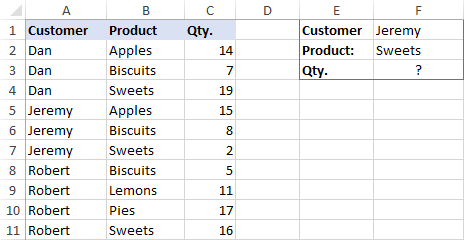A usual VLOOKUP formula won't work in this situation because it returns the first found match based on a single lookup value that you specify.

To overcome this, you can add a helper column and concatenate the values from two lookup columns (Customer and Product) there. It is important that the helper column should be the leftmost column in the table array because it's where Excel VLOOKUP always searches for the lookup value.

So, add a column to the left of your table and copy the below formula across that column. This will populate the helper column with the values from columns B and C (the space character is concatenated in between for better readability):

`=B2&" "&C2`

And then, use a standard VLOOKUP formula and place both criteria in the lookup_value argument, separated with a space:

`=VLOOKUP("Jeremy Sweets", A2:D11, 4, FALSE)`

Or, input the criteria in separate cells (G1 and G2 in our case) and concatenate those cells:

`=VLOOKUP(G1&" "&G2, A2:D11, 4, FALSE)`

As we want to return a value from column D, which is fourth in the table array, we use 4 for col_index_num. The range_lookup argument is set to FALSE to Vlookup an exact match. The screenshot below shows the result: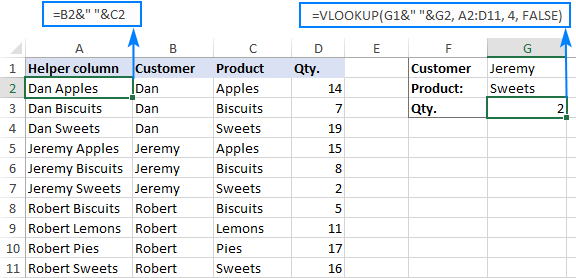In case your lookup table is in another sheet, include the sheet's name in your VLOOKUP formula. For example:

`=VLOOKUP(G1&" "&G2, Orders!A2:D11, 4, FALSE)`

Alternatively, create a named range for the lookup table (say, Orders) to make the formula easier-to-read:

`=VLOOKUP(G1&" "&G2, Orders, 4, FALSE)`

For more information, please see How to Vlookup from another sheet in Excel.

Note. For the formula to work correctly, the values in the helper column should be concatenated exactly the same way as in the lookup_value argument. For example, we used a space character to separate the criteria in both the helper column (B2&" "&C2) and VLOOKUP formula (G1&" "&G2).

### Formula 2. Excel VLOOKUP with multiple conditions

In theory, you can use the above approach to Vlookup more than two criteria. However, there are a couple of caveats. Firstly, a lookup value is limited to 255 characters, and secondly, the worksheet's design may not allow adding a helper column.

Luckily, Microsoft Excel often provides more than one way to do the same thing. To Vlookup multiple criteria, you can use either an INDEX MATCH combination or the XLOOKUP function recently introduced in Office 365.

For example, to look up based on 3 different values (Date, Customer name and Product), use one of the following formulas:

`=INDEX(D2:D11, MATCH(1, (G1=A2:A11) * (G2=B2:B11) * (G3=C2:C11), 0))`

`=XLOOKUP(1, (G1=A2:A11) * (G2=B2:B11) * (G3=C2:C11), D2:D11)`

Where:

• G1 is criteria 1 (date)
• G2 is criteria 2 (customer name)
• G3 is criteria 3 (product)
• A2:A11 is lookup range 1 (dates)
• B2:B11 is lookup range 2 (customer names)
• C2:C11 is lookup range 3 (products)
• D2:D11 is the return range (quantity)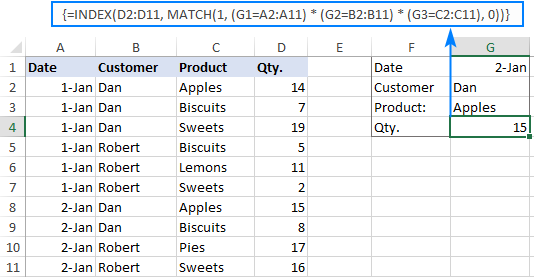Note. In all versions except Excel 365, INDEX MATCH should be entered as an CSE array formula by pressing Ctrl + Shift + Enter. In Excel 365 that supports dynamic arrays it also works as a regular formula.

For the detailed explanation of the formulas, please see:

## How to use VLOOKUP to get 2nd, 3rd or nth match

As you already know, Excel VLOOKUP can fetch only one matching value, more precisely, it returns the first found match. But what if there are several matches in your lookup array and you want to get the 2nd or 3rd instance? The task sounds quite intricate, but the solution does exist!

### Formula 1. Vlookup Nth instance

Suppose you have customer names in one column, the products they purchased in another, and you are looking to find the 2nd or 3rd product bought by a given customer.

The simplest way is to add a helper column to the left of the table like we did in the first example. But this time, we will populate it with customer names and occurrence numbers like "John Doe1", "John Doe2", etc.

To get the occurrence, use the COUNTIF function with a mixed range reference (the first reference is absolute and the second is relative like \$B\$2:B2). Since the relative reference changes based on a position of the cell where the formula is copied, in row 3 it will become \$B\$2:B3, in row 4 - \$B\$2:B4, and so on.

Concatenated with the customer name (B2), the formula takes this form:

`=B2&COUNTIF(\$B\$2:B2, B2)`

The above formula goes to A2, and then you copy it down to as many cells as needed.

After that, input the target name and occurrence number in separate cells (F1 and F2), and use the below formula to Vlookup a specific occurrence:

`=VLOOKUP(F1&F2, A2:C11, 3, FALSE)`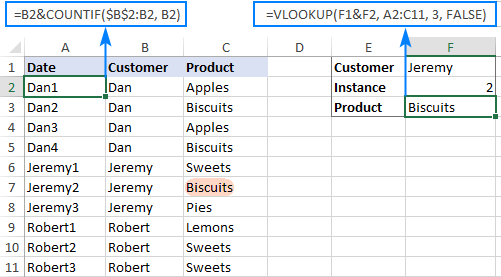### Formula 2. Vlookup 2nd occurrence

If you are looking for the 2nd instance of the lookup value, then you can do without the helper column. Instead, create the table array dynamically by using the INDIRECT function together with MATCH:

`=VLOOKUP(E1, INDIRECT("A"&(MATCH(E1, A2:A11, 0)+2)&":B11"), 2, FALSE)`

Where:

• E1 is the lookup value
• A2:A11 is the lookup range
• B11 is the last (bottom-right) cell of the lookup table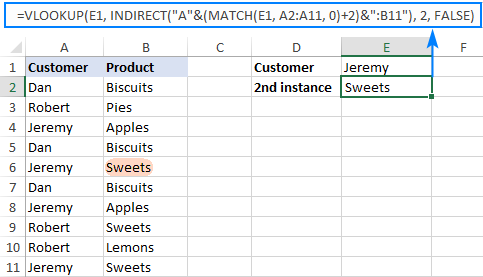Please note that the above formula is written for a specific case where data cells in the lookup table begin in row 2. If your table is somewhere in the middle of the sheet, use this universal formula, where A1 is the top-left cell of the lookup table containing a column header:

`=VLOOKUP(E1, INDIRECT("A"&(MATCH(E1, A2:A11, 0)+1+ROW(A1))&":B11"), 2, FALSE)`

#### How this formula works

Here is the key part of the formula that creates a dynamic vlookup range:

`INDIRECT("A"&(MATCH(E1, A2:A11, 0)+2)&":B11")`

The MATCH function configured for exact match (0 in the last argument) compares the target name (E1) against the list of names (A2:A11) and returns the position of the first found match, which is 3 in our case. This number is going to be used as the starting row coordinate for the vlookup range, so we add 2 to it (+1 to exclude the first instance and +1 to exclude row 1 with the column headers). Alternatively, you can use 1+ROW(A1) to calculate the necessary adjustment automatically based on the position of the header row (A1 in our case).

As the result, we get the following text string, which INDIRECT converts to a range reference:

`INDIRECT("A"&5&":B11") -> A5:B11`

This range goes to the table_array argument of VLOOKUP forcing it to start searching in row 5, leaving out the first instance of the lookup value:

`VLOOKUP(E1, A5:B11, 2, FALSE)`

## How to Vlookup and return multiple values in Excel

The Excel VLOOKUP function is designed to return just one match. Is there a way to Vlookup multiple instances? Yes, there is, though not an easy one. This requires a combined use of several functions such as INDEX, SMALL and ROW is an array formula.

For example, the below can find all occurrences of the lookup value F2 in the lookup range B2:B16 and return multiple matches from column C:

`{=IFERROR(INDEX(\$C\$2:\$C\$11, SMALL(IF(\$F\$1=\$B\$2:\$B\$11, ROW(\$C\$2:\$C\$11)-1,""), ROW()-1)),"")}`

There are 2 ways to enter the formula in your worksheet:

1. Type the formula in the first cell, press Ctrl + Shift + Enter, and then drag it down to a few more cells.
2. Select several adjacent cells in a single column (F1:F11 in the screenshot below), type the formula and press Ctrl + Shift + Enter to complete it.

Either way, the number of cells in which you enter the formula should be equal to or larger than the maximum number of possible matches.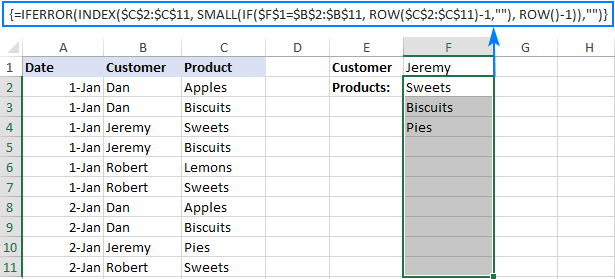For the detailed explanation of the formula logic and more examples, please see How to VLOOKUP multiple values in Excel.

## How to Vlookup in rows and columns (two-way lookup)

Two-way lookup (aka matrix lookup or 2-dimentional lookup) is a fancy word for looking up a value at the intersection of a certain row and column. There are a few different ways to do two-dimensional lookup in Excel, but since the focus of this tutorial is on the VLOOKUP function, we will naturally use it.

For this example, we'll take the below table with monthly sales and work out a VLOOKUP formula to retrieve the sales figure for a specific item in a given month.

With item names in A2:A9, month names in B1:F1, the target item in I1 and the target month in I2, the formula goes as follows:

`=VLOOKUP(I1, A2:F9, MATCH(I2, A1:F1, 0), FALSE)`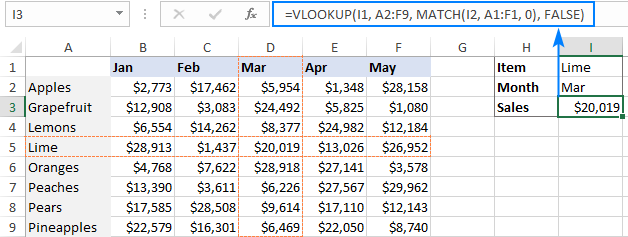### How this formula works

The core of the formula is the standard VLOOKUP function that searches for an exact match to the lookup value in I1. But since we do not know in which exactly column the sales for a specific month are, we cannot supply the column number directly to the col_index_num argument. To find that column, we use the following MATCH function:

`MATCH(I2, A1:F1, 0)`

Translated into English, the formula says: look up the I2 value in A1:F1 and return its relative position in the array. By supplying 0 to the 3rd argument, you instruct MATCH to find the value exactly equal to the lookup value (it's like using FALSE for the range_lookup argument of VLOOKUP).

Since Mar is in the 4th column in the lookup array, the MATCH function returns 4, which goes directly to the col_index_num argument of VLOOKUP:

`VLOOKUP(I1, A2:F9, 4, FALSE)`

Please pay attention that although the month names start in column B, we use A1:I1 for the lookup array. This is done in order for the number returned by MATCH to correspond to the column's position in table_array of VLOOKUP.

To learn more ways to perform matrix lookup in Excel, please see INDEX MATCH MATCH and other formulas for 2-dimensional lookup.

## How to do multiple Vlookup in Excel (nested Vlookup)

Sometimes it may happen that your main table and lookup table do not have a single column in common, which prevents you from doing a Vlookup between two tables. However, there exists another table, which does not contain the information you are looking for but has one common column with the main table and another common column with the lookup table.

In below image illustrates the situation: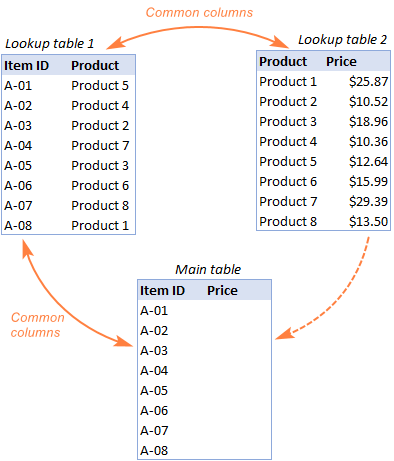The goal is to copy prices to the main table based on Item IDs. The problem is that the table containing prices does not have the Item IDs, meaning we will have to do two Vlookups in one formula.

For the sake of convenience, let's create a couple of named ranges first:

• Lookup table 1 is named Products (D3:E10)
• Lookup table 2 is named Prices (G3:H10)

The tables can be in the same or different worksheets.

And now, we will perform the so-called double Vlookup, aka nested Vlookup.

First, make a VLOOKUP formula to find the product name in the Lookup table 1 (named Products) based on the item id (A3):

`=VLOOKUP(A3, Products, 2, FALSE)`

Next, put the above formula in the lookup_value argument of another VLOOKUP function to pull prices from Lookup table 2 (named Prices) based on the product name returned by the nested VLOOKUP:

`=VLOOKUP(VLOOKUP(A3, Products, 2, FALSE), Prices, 2, FALSE)`

The screenshot below shows our nested Vlookup formula in action: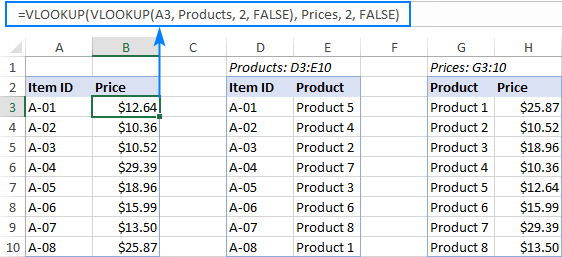## How to Vlookup multiple sheets dynamically

Sometimes, you may have data in the same format split over several worksheets. And your aim is to pull data from a specific sheet depending on the key value in a given cell.

This may be easier to understand from an example. Let's say, you have a few regional sales reports in the same format, and you are looking to get the sales figures for a specific product in certain regions: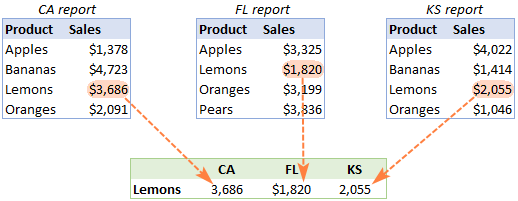Like in the previous example, we start with defining a few names:

• Range A2:B5 in CA sheet is named CA_Sales.
• Range A2:B5 in FL sheet is named FL_Sales.
• Range A2:B5 in KS sheet is named KS_Sales.

As you can see, all the named ranges have a common part (Sales) and unique parts (CA, FL, KS). Please be sure to name your ranges in a similar manner as it's essential for the formula we are going to build.

### Formula 1. INDIRECT VLOOKUP to dynamically pull data from different sheets

If your task is to retrieve data from multiple sheets, a VLOOKUP INDIRECT formula is the best solution – compact and easy-to-understand.

For this example, we organize the summary table in this way:

• Input the products of interest in A2 and A3. Those are our lookup values.
• Enter the unique parts of the named ranges in B1, C1 and D1.

And now, we concatenate the cell containing the unique part (B1) with the common part ("_Sales"), and feed the resulting string to INDIRECT:

`INDIRECT(B\$1&"_Sales")`

The INDIRECT function transforms the string into a name that Excel can understand, and you put it in the table_array argument of VLOOKUP:

`=VLOOKUP(\$A2, INDIRECT(B\$1&"_Sales"), 2, FALSE)`

The above formula goes to B2, and then you copy it down and to the right.

Please pay attention that, in the lookup value (\$A2), we've locked the column coordinate with absolute cell reference so that the column remains fixed when the formula is copied to the right. In the B\$1 reference, we locked the row because we want the column coordinate to change and supply an appropriate name part to INDIRECT depending on the column into which the formula is copied: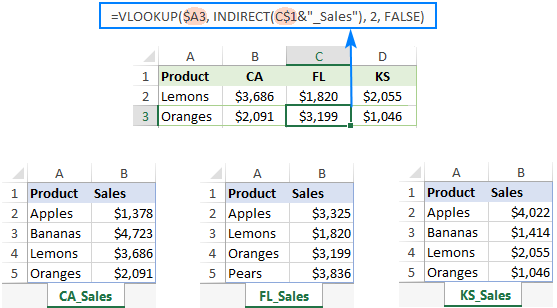If your main table is organized differently, the lookup values in a row and unique parts of the range names in a column, then you should lock the row coordinate in the lookup value (B\$1) and the column coordinate in the name parts (\$A2):

`=VLOOKUP(B\$1, INDIRECT(\$A2&"_Sales"), 2, FALSE)`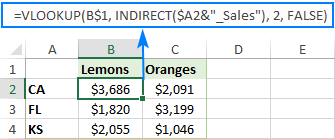### Formula 2. VLOOKUP and nested IFs to look up multiple sheets

In situation when you have just two or three lookup sheets, you can use a fairly simple VLOOKUP formula with nested IF functions to select the correct sheet based on the key value in a particular cell:

`=VLOOKUP(\$A2, IF(B\$1="CA", CA_Sales, IF(B\$1="FL", FL_Sales, IF(B\$1="KS", KS_Sales,""))), 2, FALSE)`

Where \$A2 is the lookup value (item name) and B\$1 is the key value (state):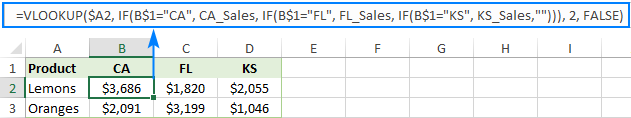In this case, you do not necessarily need to define names and can use external references to refer to another sheet or workbook.

For more formula examples, please see How to VLOOKUP across multiple sheets in Excel.

That's how to use VLOOKUP in Excel. I thank you for reading and hope to see you on our blog next week!